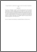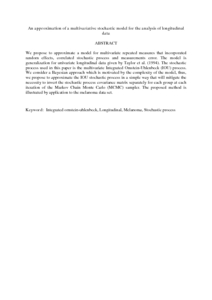# An approximation of a multivariative stochastic model for the analysis of longitudinal data

## Citation

Abu Bakar, Mohd Rizam and Ali Salah, Khalid (2008) An approximation of a multivariative stochastic model for the analysis of longitudinal data. European Journal of Scientific Research, 20 (1). pp. 76-87. ISSN 1450-216X

## Abstract

We propose to approximate a model for multivariate repeated measures that incorporated random effects, correlated stochastic process and measurements error. The model is generalization for univariate longitudinal data given by Taylor et al. (1994). The stochastic process used in this paper is the multivariate Integrated Omstein-Uhlenbeck (IOU) process. We consider a Bayesian approach which is motivated by the complexity of the model, thus, we propose to approximate the IOU stochastic process in a simple way that will mitigate the necessity to invert the stochastic process covariance matrix separately for each group at each iteration of the Markov Chain Monte Carlo (MCMC) sampler. The proposed method is illustrated by application to the melanoma data set.Preview
PDF (Abstract)
An approximation of a multivariative stochastic model for the analysis of longitudinal data.pdfView Item